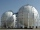Body volume + spherical cap - examples

1. Spherical capFrom the sphere of radius 18 was truncated spherical cap. Its height is 12. What part of the volume is spherical cap from whole sphere?
2. Spherical segmentSpherical segment with height h=6 has a volume V=134. Calculate the radius of the sphere of which is cut this segment.A domed stadium is in the shape of spherical segment with a base radius of 150 m. The dome must contain a volume of 3500000 m³. Determine the height of the dome at its centre to the nearest tenth of a meter.The vessel hemispherical hollow is filled with water to a height of 10 cm =. How many liters of water are inside if the inside diameter of the hollow is d = 28cm?The tank of a water tower is a sphere of radius 35ft. If the tank is filled to one quarter of full, what is the height of the water?